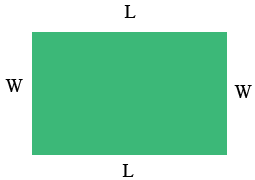SEARCH HOMEMath Central Quandaries & QueriesQuestion from Ash: I need to know how to figure out the dimensions of a rectangle. I know that the area is 30ft and the perimeter is 26ft. I'm 15 and I have no idea what I'm supposed to do.Hi Ash,

I would first draw a diagramThe dimensions of a rectangle are its length and its width. I used $L$ to designate the length and $W$ to designate its width.

The area of a rectangle is its length times its width so

$L \times W = 30 \mbox{ square feet}$

and its perimeter is the distance all the way around so

$L + W + L + W = 26 \mbox{ feet.}$

Hence the distance half way around is

$L + W = 13 \mbox{ feet.}$

Thus you have two equations

$L \times W = 30$

and

$L + W + 13.$

Do you know two numbers with a sum of 13 and a product of 30?

HarleyMath Central is supported by the University of Regina and The Pacific Institute for the Mathematical Sciences.